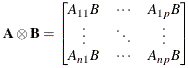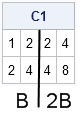There are many ways to multiply scalars, vectors, and matrices, but the Kronecker product (also called the direct product) is multiplication on steroids.

The Kronecker product looks scary, but it is actually simple. The Kronecker product is merely a way to pack multiples of a matrix B into a block matrix. If A is an n x p matrix, then the direct product A@B is the block matrix formed by stacking copies of B into the shape of A and multiplying the (i,j)th block by Aij. In symbols:In SAS software, the Kronecker product is available in the SAS/IML matrix language. The direct product operator in SAS/IML is represented by using the "at sign" (@) operator.

The first matrix in the Kronecker product determines the shape of the final (block) matrix. The following example is equivalent to the horizontal concatenation of B and 2B:

```proc iml; B = {1 2, 2 4}; A = {1 2}; /* dimension of A determines shape of block matrix */ C1 = A@B; /* 1*B || 2*B */ print C1;```The first matrix can be any shape. To obtain a product that is a block-diagonal matrix, you can form the Kronecker product of a diagonal matrix with another matrix, as follows:

```A = {2 0, 0 4}; /* = diag({2 4}) */ C2 = A@B; /* = block(2*B, 4*B) */ print C2;```Block-diagonal matrices are used in mixed models. The Kronecker product makes it easy to construct block matrices where each block is a multiple of a matrix B.

Notice that the product A@B is very simple if A is a matrix of zeros and ones. In that case, the Kronecker product creates a block matrix where each block is either a copy of B or a zero block. For example, execute the statement C3 = I(3)@B to form a block diagonal matrix that contains three copies of B.

In the early days of the SAS/IML language, the Kronecker product was used a lot. Prior to SAS version 8, matrices had to be the same dimension in order to add or subtract them. The Kronecker product can be used to coerce a vector into a matrix shape, and the Kronecker operator appears in graduate-level textbooks about matrix operations in statistics. For example, suppose that you want to center a data matrix by subtracting the mean of each column. A modern SAS/IML program would use the following shorthand expression to center the matrix:

```/* read data matrix into X */ use Sashelp.Class; read all var _num_ into X; close Sashelp.Class; mean = x[:,]; /* vector contains mean of each column */ CenterX = X - mean; /* modern code: Subtract the vector */```

In contrast, a SAS/IML program that was written in the 1980s or '90s would use the Kronecker product with a vectors of 1s to create a matrix that is the same shape as X. Each row of the resulting matrix is a copy of the mean vector. Here's how an old program (or a textbook) might express the operation that centers a data matrix:

```/* Old code: expand meanX into matrix that is same shape as X */ CenterX = X - mean @ j(nrow(X),1,1); /* subtract matrices of same dim */```